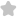1. Let f(0) = sin( tan" someo ), wh...
Question# 1. Let f(0) = sin( tan" someo ), where JEE/Engineering Exams Maths SolutionShare964.0 (1 ratings)( f(theta)=sin left(tan ^{-1} frac{sin theta}{sqrt{cos 2 theta}}right) )964.0 (1 ratings) Rate SolutionShareQuick and Stepwise Solutions Just click and SendOVER 20 LAKH QUESTIONS ANSWERED Download App for Free